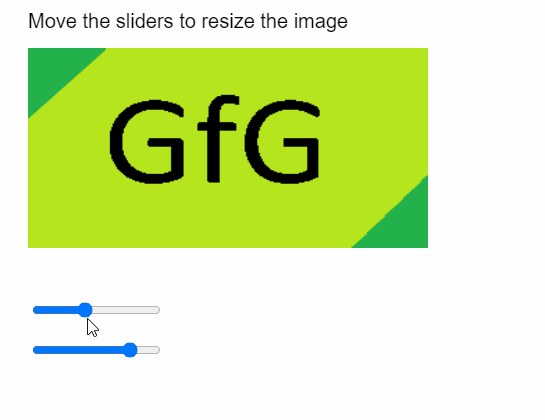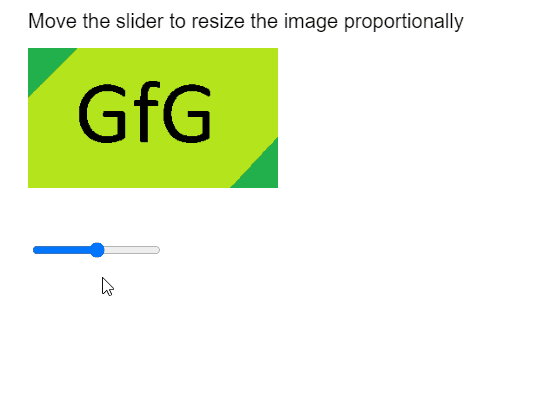GeeksforGeeks App
Open AppBrowser
Continue

## Related Articles

The resize() method of p5.Image in p5.js is used to resize the image to the given width and height. The image can be scaled proportionally by using 0 as one of the values of the width and height.

Syntax:

```resize( width, height )
```

Parameters: This function accepts two parameters as mentioned above and described below.

• width: It is a number that specifies the width of the resized image.
• height: It is a number that specifies the height of the resized image.

The examples below illustrate the resize() method in p5.js:

Example 1:

## Javascript

 `function` `preload() {``    ``img_orig = loadImage(``"sample-image.png"``);``}`` ` `function` `setup() {``    ``createCanvas(500, 400);``    ``textSize(20);`` ` `    ``heightSlider =``      ``createSlider(0, 500, 200);``    ``heightSlider.position(30, 300);`` ` `    ``widthSlider =``      ``createSlider(0, 500, 400);``    ``widthSlider.position(30, 340);``}`` ` `function` `draw() {``    ``clear();`` ` `    ``text(``"Move the sliders to resize the image"``,``      ``20, 20);``    ``image(img_orig, 20, 40);`` ` `    ``new_height = heightSlider.value();``    ``new_width = widthSlider.value();``     ` `    ``img_orig.resize(new_width, new_height);``}`

Output:Example 2:

## Javascript

 `function` `preload() {``    ``img_orig = loadImage(``"sample-image.png"``);``}`` ` `function` `setup() {``    ``createCanvas(500, 400);``    ``textSize(20);`` ` `    ``sizeSlider =``      ``createSlider(0, 500, 250);``    ``sizeSlider.position(30, 240);``}`` ` `function` `draw() {``    ``clear();`` ` `    ``text(``"Move the slider to resize "` `+``      ``"the image proportionally"``, 20, 20);``    ``image(img_orig, 20, 40);`` ` `    ``new_size = sizeSlider.value();``     ` `    ``// Setting one of the values as 0,``    ``// for proportional resizing``    ``img_orig.resize(new_size, 0);``}`

Output:Online editor: https://editor.p5js.org/
Environment Setup: https://www.geeksforgeeks.org/p5-js-soundfile-object-installation-and-methods/
Reference: https://p5js.org/reference/#/p5.Image/resize

My Personal Notes arrow_drop_up
Related Tutorials